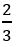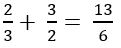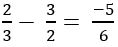Welcome To Basics In Maths

# Trigonometric Ratios(Qns.& Ans) V.S.A.Q.’STrigonometric Ratios(Qns.& Ans) V.S.A.Q.’S designed by the ‘Basics in Maths‘ team.These notes to do help the intermediate First-year Maths students.

Inter Maths – 1A two marks questions and solutions are very useful in I.P.E examinations.

## Trigonometric Ratios Up to Transformations

Question 1

Find the value of sin2(π/10) + sin2(4π/10) + sin2(6π/10) + sin2(9π/10)

Sol:

sin2(π/10) + sin2(4π/10) + sin2(6π/10) + sin2(9π/10)

= sin2(π/10) + sin2(π/2 – π/10) + sin2(π/2+ π/10) + sin2(π – π/10)

= sin2(π/10) + cos2(π/10) + cos2(π/10) + sin2(π/10)

= 1 + 1 = 2

Question 2

If sin θ = 4/5 and θ not in the first quadrant, find the value of cos θ

Sol:

Given sin θ = 4/5 and θ not in the first quadrant

⇒ θ in the second quadrant

⇒ cos θ < 0

cos2θ = 1 – sin2 θ

=1 – (4/5)2

= 1 – 16/25

∴cos θ   = – 3/5 (∵cos θ < 0)

Question 3

If 3sin θ + 4 cos θ = 5, then find the value of 4 sin θ – 3cos θ

Sol:

Given, 3sin θ + 4 cos θ = 5

let 4 sin θ – 3cos θ = x

(3sin θ + 4 cos θ )2 + (4 sin θ – 3cos θ)2 = 52 + x2

9 sin2 θ + 16 cos2 θ + 12 sin θ cos θ + 16 sin2 θ + 9 cos2 θ – 12sin θ cis θ = 25 + x2

25 sin2 θ + 25 cos2 θ = 25 + x2

25 = 25 + x2

⇒ x2 = 0

x = 0

∴ 4 sin θ – 3cos θ = 0

#### Question 4

If sec θ + tan θ =, find the value of sin θ and determine the quadrant in which θ lies

Sol:

Given, sec θ + tan θ =  ———— (1)

We know that sec2 θ – tan2 θ = 1

⇒ (sec θ + tan θ) (sec θ – tan θ) = 1

(1) + (2)

⇒ (sec θ + tan θ) + (sec θ – tan θ) =(1) – (2)

⇒ (sec θ + tan θ) – (sec θ – tan θ) =Since sec θ positive and tan θ is negative θ lies in the 4th quadrant.

Question 5

Prove that cot (π/16). cot (2π/16). cot (3π/16).… cot (7π/16) = 1

Sol:

cot (π/16). cot (2π/16). cot (3π/16).… cot (7π/16)

= cot (π/16). cot (2π/16). cot (3π/16). cot (4π/16). cot (5π/16) cot (6π/16) cot (7π/16)

= cot (π/16). cot (2π/16). cot (3π/16). cot (π/4). cot (π/2 – 3π/16) cot (π/2 – 2π/16) cot (π/2 – π/16)

= cot (π/16). cot (2π/16). cot (3π/16). cot (π/4). tan (3π/16) tan (2π/16) tan (π/16)

= [cot (π/16). tan (π/16)] [cot (2π/16). tan (2π/16)] [cot (3π/16). tan (3π/16]. cot (π/4)

= 1.1.1.1

=1